## Henry’s Law

### Learning Objectives

• State Henry’s Law.
• Perform calculations involving Henry’s Law.

### How is sipping soda in outer space different then on Earth?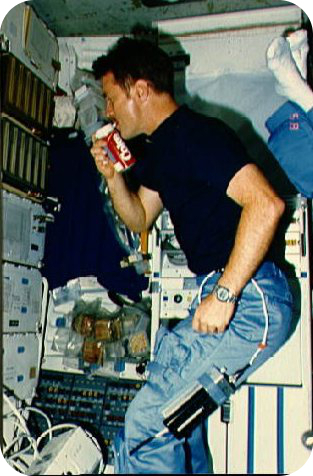Astronaut drinks out of a specially-designed can. Courtesy of NASA.

Having a soft drink in outer space poses some special problems. Under microgravity, the carbonation could quickly dissipate if not kept under pressure. You can’t open the can or you will lose carbonation. So a special pressurized container has been developed to get around the problem of gas loss at low gravity.

## Henry’s Law

Pressure has very little effect on the solubility of solids or liquids, but has a significant effect on the solubility of gases. Gas solubility increases as the partial pressure of a gas above the liquid increases. Suppose a certain volume of water is in a closed container with the space above it occupied by carbon dioxide gas at standard pressure. Some of the CO 2 molecules come into contact with the surface of the water and dissolve into the liquid. Now suppose that more CO 2 is added to the space above the container, causing a pressure increase. More CO 2 molecules are now in contact with the water and so more of them dissolve. Thus the solubility increases as the pressure increases. As with a solid, the CO 2 that is undissolved reaches an equilibrium with the dissolved CO2 , represented by the following equation.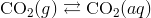At equilibrium, the rate of gaseous CO2 dissolving is equal to the rate of dissolved CO2 coming out of the solution.

When carbonated beverages are packaged, they are done so under high CO2 pressure so that a large amount of carbon dioxide dissolves in the liquid. When the bottle is open, the equilibrium is disrupted because the CO2 pressure above the liquid decreases. Immediately, bubbles of CO2 rapidly exit the solution and escape out of the top of the open bottle. The amount of dissolve CO2 decreases. If the bottle is left open for an extended period of time, the beverage becomes “flat” as more and more CO2 comes out of the liquid.

The relationship of gas solubility to pressure is described by Henry’s law, named after English chemist William Henry (1774-1836). Henry’s Law states that the solubility of a gas in a liquid is directly proportional to the partial pressure of the gas above the liquid. Henry’s law can be written as follows: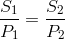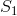andare the solubility and the pressure at an initial set of conditions;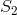and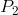are the solubility and pressure at another changed set of conditions. Solubilities of gases are typically reported in g/L, as seen in sample problem 16.1.

### Sample Problem:

The solubility of a certain gas in water is 0.745 g/L at standard pressure. What is its solubility when the pressure above the solution is raised to 4.50 atm? The temperature is constant at 20°C.

Step 1: List the known quantities and plan the problem.

Known

•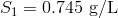•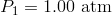•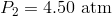Unknown

•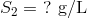Substitute into Henry’s law and solve for.

Step 2: Solve.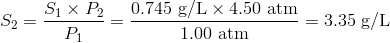Step 3: Think about your result.

The solubility is increased to 4.5 times its original value according to the direct relationship.

### Summary

• The pressure above a liquid affects the solubility of a gas in the liquid.

### Practice

Watch the video at the link below and answer the questions:

http://www.youtube.com/watch?v=eM56JYel1aM

1. What affects the Henry’s law constant?
2. What do you need to be careful of in the way of units?
3. If we know the atmospheric pressure and the mole fraction of the gas, how do we calculate the partial pressure of the gas?

### Review

1. Does pressure affect the solubility of liquids and solids?
2. If you increase the pressure of a gas above the liquid, how does that affect the amount of gas dissolved in the liquid?
3. What equilibrium is eventually reached?

## Glossary

• Henry’s Law: The solubility of a gas in a liquid is directly proportional to the partial pressure of the gas above the liquid.